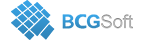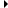BCGControlBar Pro for MFC
CBCGPChartBaseFormulaabstract

## Detailed Description

The CBCGPChartBaseFormula class is a base abstract class for series data transition and trend line support. It also manages an array of input series and stores user defined data, which later is passed to custom callbacks.

Series transition allows to take one or more input series, apply some transition formula (like sum, difference, average etc) to their data and display a result on the diagram as a new series. The basic formulas have been implemented by CBCGPChartTransitionFormula. It also accepts a pointer to a custom callback, which allows to implement custom calculations.

Trend line is a (parent) series connected with a CBCGPChartTrendFormula object and accepting one input series. The data in the series is processed to generate coefficients for linear, exponential, logarithmic, power and polynomial (with 2- 6 range power) trend lines. The parent series does not contain any data points. Given these coefficients it calculates screen points for a non-discrete trend line curve to be displayed on the diagram. You can also specify some custom coefficients (the input series can be NULL in this case) and display one of the listed above trend line types on the diagram.

You should always call CBCGPChartSeries::SetFormula to connect a formula with a series. When a formula object has been set, it's invalidated and copied into internal formula object. Use CBCGPChartSeries::GetFormula for future access to the formula object.

CBCGPChartTransitionFormula, CBCGPChartTrendFormulaInheritance diagram for CBCGPChartBaseFormula:

## Public Member Functions

FindInputSeriesIndexFinds input series index.

GeneratePointsGenerates points (data points or screen points) that represent the formula on chart diagram.

GetInputSeriesAtReturns an input series located at the specified position.

GetInputSeriesCountReturns the number of input series.

GetLParamReturns user-defined data.

GetParentSeriesReturns a pointer to a parent series.

IsNonDiscreteCurveTells whether the formula is displayed as a non-discrete curve.

SetLParamSets user-defined data.

SetParentSeriesSets a parent series that represents a formula on the diagram.